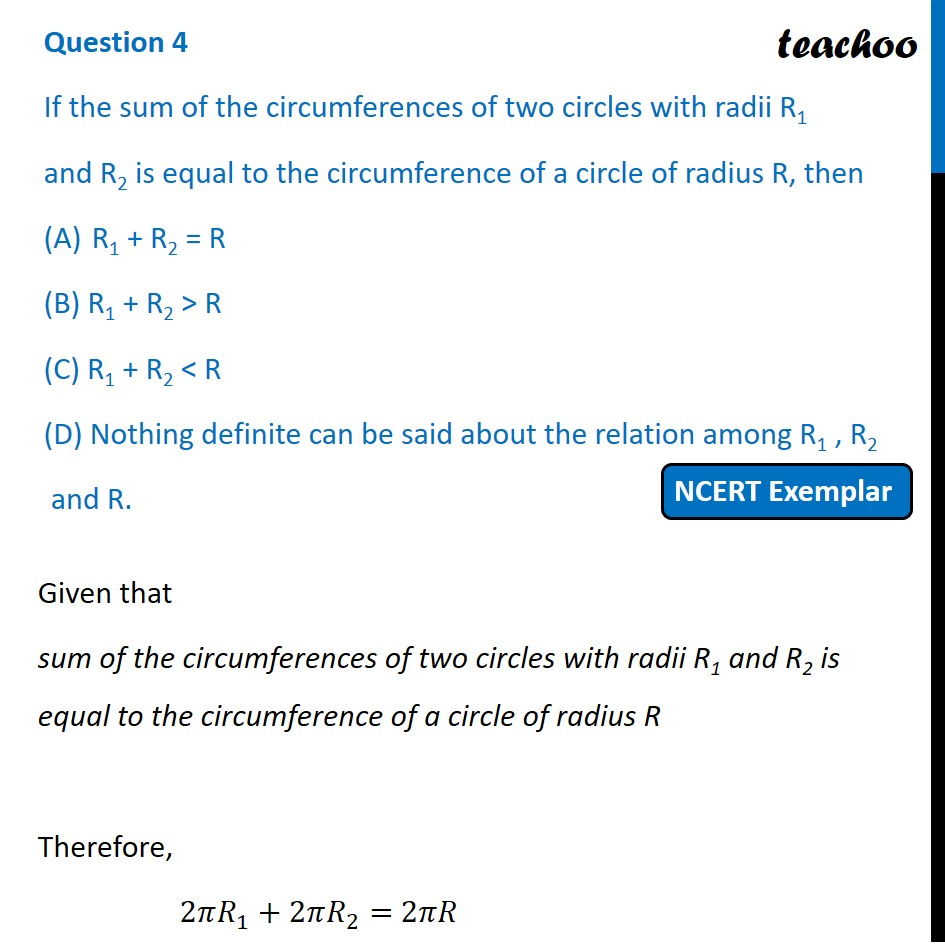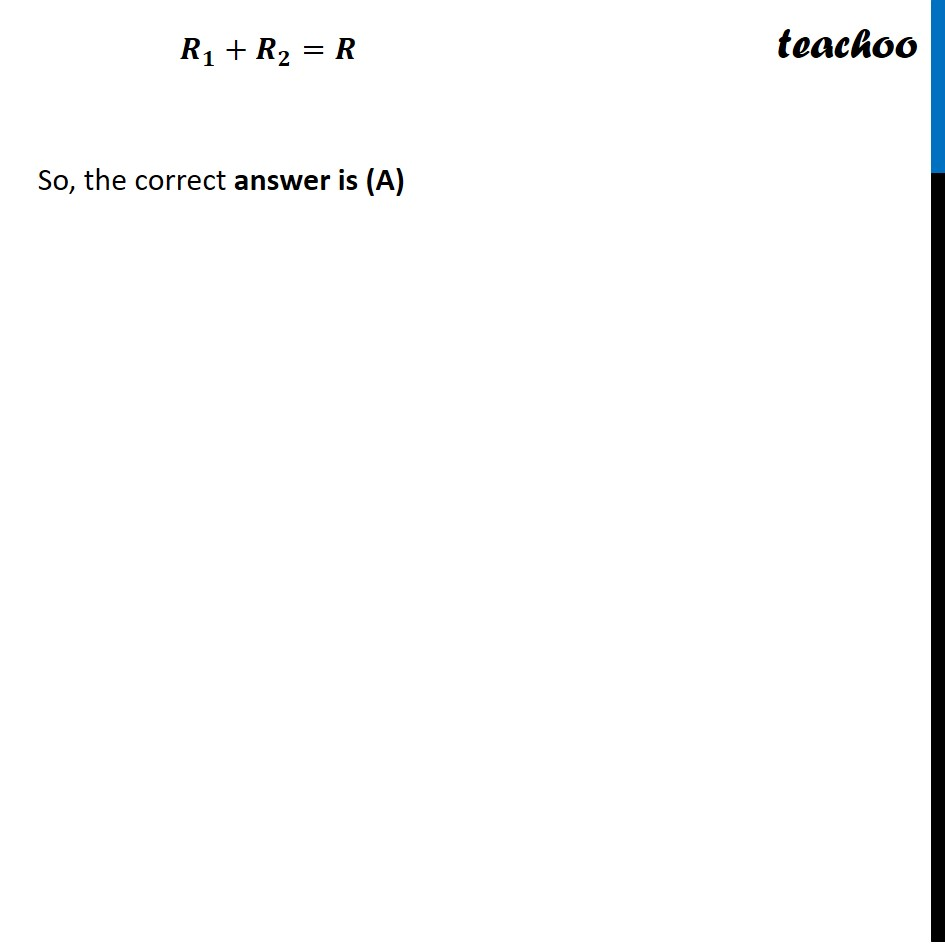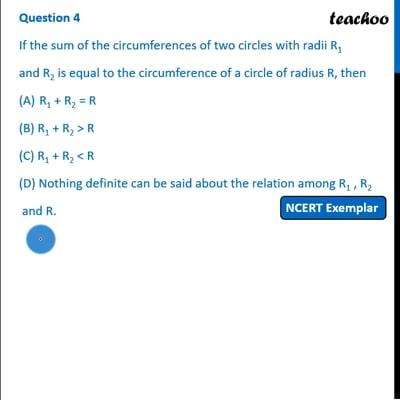NCERT Exemplar - MCQ

Chapter 12 Class 10 Areas related to Circles
Serial order wise

## (D) Nothing definite can be said about the relation among R 1 , R 2 and R.This video is only available for Teachoo black users

Get live Maths 1-on-1 Classs - Class 6 to 12

### Transcript

Question 4 If the sum of the circumferences of two circles with radii R1 and R2 is equal to the circumference of a circle of radius R, then R1 + R2 = R (B) R1 + R2 > R (C) R1 + R2 < R (D) Nothing definite can be said about the relation among R1 , R2 and R. Given that sum of the circumferences of two circles with radii R1 and R2 is equal to the circumference of a circle of radius R Therefore, 2𝜋𝑅_1+2𝜋𝑅_2= 2𝜋𝑅 𝑹_𝟏+𝑹_𝟐=𝑹 So, the correct answer is (A)